# (0,5,6,7,11) using: Implement the circuit defined by equation F(a,b,c,d) 1. 4-to-1 multiplexers and logic gates. 2....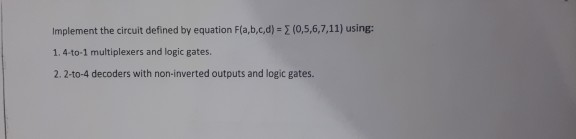(0,5,6,7,11) using: Implement the circuit defined by equation F(a,b,c,d) 1. 4-to-1 multiplexers and logic gates. 2. 2-to-4 decoders with non-inverted outputs and logic gates.The truth table for this: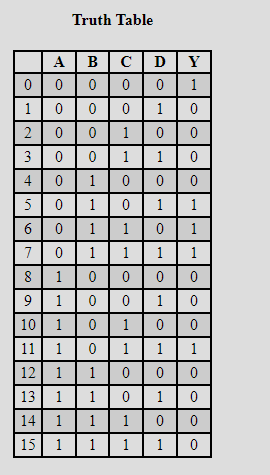K-- map of this: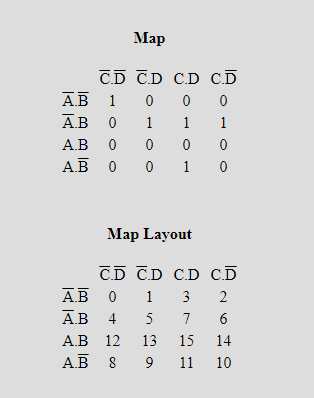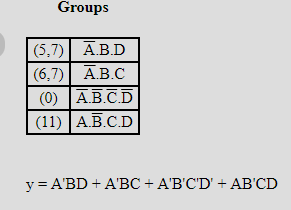Put S0 = A, And S1 = B

The truth table for S0 and  S1

 S0 S1 Y 0 0 D0 0 1 D1 1 0 D3 1 1 D2

Use MUX 4X1 :

D0 = C'.D'

D1 = C + D

D2 = 0 (because no pair form in this k- map)

D3 = C.D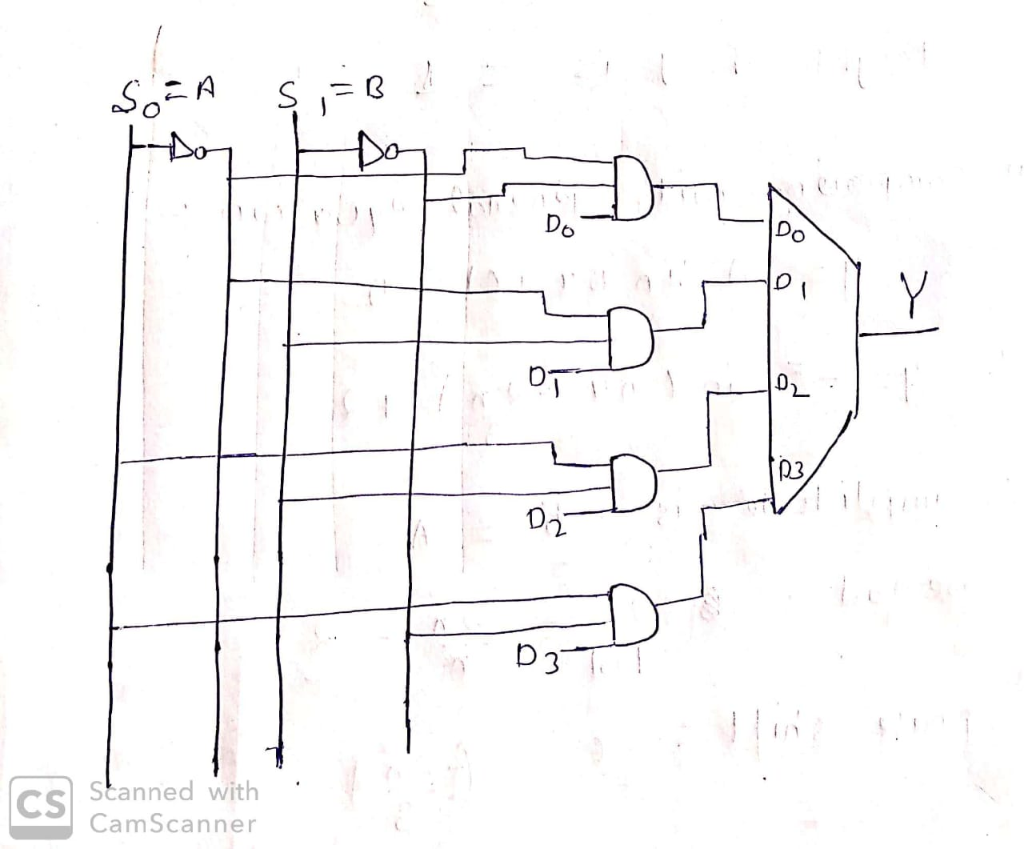For any doubt drop a comment.

Thank you!

##### Add Answer of: (0,5,6,7,11) using: Implement the circuit defined by equation F(a,b,c,d) 1. 4-to-1 multiplexers and logic gates. 2....
More Homework Help Questions Additional questions in this topic.